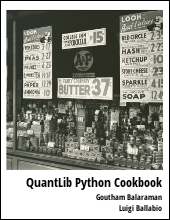Provides an introduction to constructing implied volatility surface consistend with the smile observed in the market and calibrating Heston model using QuantLib Python.

Visit here for other QuantLib Python examples. If you found these posts useful, please take a minute by providing some feedback.

European options on an equity underlying such as an index (S&P 500) or a stock (AMZN) trade for different combinations of strikes and maturities. It turns out that the Black-Scholes implied volatility for these options with different maturities and strikes is not the same. The fact that the implied volatility varies with strike is often referred in the market as having a smile.

In :
import QuantLib as ql
import math


First let us define some of the basic data conventions such as the day_count, calendar etc.

In :
day_count = ql.Actual365Fixed()
calendar = ql.UnitedStates()

calculation_date = ql.Date(6, 11, 2015)

spot = 659.37
ql.Settings.instance().evaluationDate = calculation_date

dividend_yield = ql.QuoteHandle(ql.SimpleQuote(0.0))
risk_free_rate = 0.01
dividend_rate = 0.0
flat_ts = ql.YieldTermStructureHandle(
ql.FlatForward(calculation_date, risk_free_rate, day_count))
dividend_ts = ql.YieldTermStructureHandle(
ql.FlatForward(calculation_date, dividend_rate, day_count))


Following is a sample matrix of volatility quote by exipiry and strike. The volatilities are log-normal volatilities and can be interpolated to construct the implied volatility surface.

In :
expiration_dates = [ql.Date(6,12,2015), ql.Date(6,1,2016), ql.Date(6,2,2016),
ql.Date(6,3,2016), ql.Date(6,4,2016), ql.Date(6,5,2016),
ql.Date(6,6,2016), ql.Date(6,7,2016), ql.Date(6,8,2016),
ql.Date(6,9,2016), ql.Date(6,10,2016), ql.Date(6,11,2016),
ql.Date(6,12,2016), ql.Date(6,1,2017), ql.Date(6,2,2017),
ql.Date(6,3,2017), ql.Date(6,4,2017), ql.Date(6,5,2017),
ql.Date(6,6,2017), ql.Date(6,7,2017), ql.Date(6,8,2017),
ql.Date(6,9,2017), ql.Date(6,10,2017), ql.Date(6,11,2017)]
strikes = [527.50, 560.46, 593.43, 626.40, 659.37, 692.34, 725.31, 758.28]
data = [
[0.37819, 0.34177, 0.30394, 0.27832, 0.26453, 0.25916, 0.25941, 0.26127],
[0.3445, 0.31769, 0.2933, 0.27614, 0.26575, 0.25729, 0.25228, 0.25202],
[0.37419, 0.35372, 0.33729, 0.32492, 0.31601, 0.30883, 0.30036, 0.29568],
[0.37498, 0.35847, 0.34475, 0.33399, 0.32715, 0.31943, 0.31098, 0.30506],
[0.35941, 0.34516, 0.33296, 0.32275, 0.31867, 0.30969, 0.30239, 0.29631],
[0.35521, 0.34242, 0.33154, 0.3219, 0.31948, 0.31096, 0.30424, 0.2984],
[0.35442, 0.34267, 0.33288, 0.32374, 0.32245, 0.31474, 0.30838, 0.30283],
[0.35384, 0.34286, 0.33386, 0.32507, 0.3246, 0.31745, 0.31135, 0.306],
[0.35338, 0.343, 0.33464, 0.32614, 0.3263, 0.31961, 0.31371, 0.30852],
[0.35301, 0.34312, 0.33526, 0.32698, 0.32766, 0.32132, 0.31558, 0.31052],
[0.35272, 0.34322, 0.33574, 0.32765, 0.32873, 0.32267, 0.31705, 0.31209],
[0.35246, 0.3433, 0.33617, 0.32822, 0.32965, 0.32383, 0.31831, 0.31344],
[0.35226, 0.34336, 0.33651, 0.32869, 0.3304, 0.32477, 0.31934, 0.31453],
[0.35207, 0.34342, 0.33681, 0.32911, 0.33106, 0.32561, 0.32025, 0.3155],
[0.35171, 0.34327, 0.33679, 0.32931, 0.3319, 0.32665, 0.32139, 0.31675],
[0.35128, 0.343, 0.33658, 0.32937, 0.33276, 0.32769, 0.32255, 0.31802],
[0.35086, 0.34274, 0.33637, 0.32943, 0.3336, 0.32872, 0.32368, 0.31927],
[0.35049, 0.34252, 0.33618, 0.32948, 0.33432, 0.32959, 0.32465, 0.32034],
[0.35016, 0.34231, 0.33602, 0.32953, 0.33498, 0.3304, 0.32554, 0.32132],
[0.34986, 0.34213, 0.33587, 0.32957, 0.33556, 0.3311, 0.32631, 0.32217],
[0.34959, 0.34196, 0.33573, 0.32961, 0.3361, 0.33176, 0.32704, 0.32296],
[0.34934, 0.34181, 0.33561, 0.32964, 0.33658, 0.33235, 0.32769, 0.32368],
[0.34912, 0.34167, 0.3355, 0.32967, 0.33701, 0.33288, 0.32827, 0.32432],
[0.34891, 0.34154, 0.33539, 0.3297, 0.33742, 0.33337, 0.32881, 0.32492]]


## Implied Volatility Surface

Each row in data is a different exipiration time, and each column corresponds to various strikes as given in strikes. We load all this data into the QuantLib Matrix object. This can then be used seamlessly in the various surface construction routines. The variable implied_vols holds the above data in a Matrix format. One unusual bit of info that one needs to pay attention to is the ordering of the rows and columns in the Matrix object. The implied volatilities in the QuantLib context needs to have strikes along the row dimension and expiries in the column dimension. This is transpose of the way the data was constructed above. All of this detail is taken care by swapping the i and j variables below. Pay attention to the line:

implied_vols[i][j] = data[j][i]


in the cell below.

In :
implied_vols = ql.Matrix(len(strikes), len(expiration_dates))
for i in range(implied_vols.rows()):
for j in range(implied_vols.columns()):
implied_vols[i][j] = data[j][i]


Now the Black volatility surface can be constructed using the BlackVarianceSurface method.

In :
black_var_surface = ql.BlackVarianceSurface(
calculation_date, calendar,
expiration_dates, strikes,
implied_vols, day_count)


The volatilities for any given strike and expiry pair can be easily obtained using black_var_surface shown below.

In :
strike = 600.0
expiry = 1.2 # years
black_var_surface.blackVol(expiry, strike)

Out:
0.3352982638587421

## Visualization

In :
import numpy as np
% matplotlib inline
from mpl_toolkits.mplot3d import Axes3D
import matplotlib.pyplot as plt
from matplotlib import cm


Given an expiry, we can visualize the volatility as a function of the strike.

In :
strikes_grid = np.arange(strikes, strikes[-1],10)
expiry = 1.0 # years
implied_vols = [black_var_surface.blackVol(expiry, s)
for s in strikes_grid] # can interpolate here
actual_data = data # cherry picked the data for given expiry

fig, ax = plt.subplots()
ax.plot(strikes_grid, implied_vols, label="Black Surface")
ax.plot(strikes, actual_data, "o", label="Actual")
ax.set_xlabel("Strikes", size=12)
ax.set_ylabel("Vols", size=12)
legend = ax.legend(loc="upper right")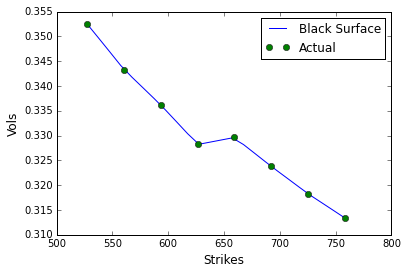The whole volatility surface can also be visualised as shown below.

In :
plot_years = np.arange(0, 2, 0.1)
plot_strikes = np.arange(535, 750, 1)
fig = plt.figure()
ax = fig.gca(projection='3d')
X, Y = np.meshgrid(plot_strikes, plot_years)
Z = np.array([black_var_surface.blackVol(y, x)
for xr, yr in zip(X, Y)
for x, y in zip(xr,yr) ]
).reshape(len(X), len(X))

surf = ax.plot_surface(X,Y,Z, rstride=1, cstride=1, cmap=cm.coolwarm,
linewidth=0.1)
fig.colorbar(surf, shrink=0.5, aspect=5)

Out:
<matplotlib.colorbar.Colorbar instance at 0x0963C7D8>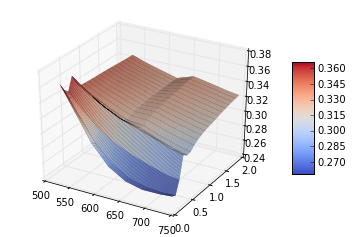One can also construct a local volatility surface (a la Dupire) using the LocalVolSurface. There are some issues with this as shown below.

In :
local_vol_surface = ql.LocalVolSurface(
ql.BlackVolTermStructureHandle(black_var_surface),
flat_ts,
dividend_ts,
spot)

In :
plot_years = np.arange(0, 2, 0.1)
plot_strikes = np.arange(535, 750, 1)
fig = plt.figure()
ax = fig.gca(projection='3d')
X, Y = np.meshgrid(plot_strikes, plot_years)
Z = np.array([local_vol_surface.localVol(y, x)
for xr, yr in zip(X, Y)
for x, y in zip(xr,yr) ]
).reshape(len(X), len(X))

surf = ax.plot_surface(X, Y, Z, rstride=1, cstride=1, cmap=cm.coolwarm,
linewidth=0.1)
fig.colorbar(surf, shrink=0.5, aspect=5)

Out:
<matplotlib.colorbar.Colorbar instance at 0x091720F8>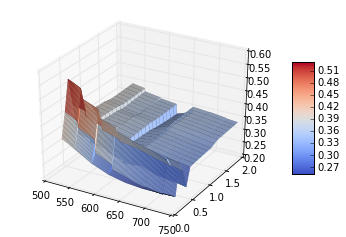The correct pricing of local volatility surface requires an arbitrage free implied volatility surface. If the input implied volatility surface is not arbitrage free, this can lead to negative transition probabilities and/or negative local volatilities and can give rise to mispricing. Refer to Fengler's arbtirage free smoothing  which QuantLib currently lacks.

When you use an arbitrary smoothing, you will notice that the local volatility surface leads to undesired negative volatilities.

In :
black_var_surface.setInterpolation("bicubic")
local_vol_surface = ql.LocalVolSurface(
ql.BlackVolTermStructureHandle(black_var_surface),
flat_ts,
dividend_ts,
spot)
plot_years = np.arange(0, 2, 0.15)
plot_strikes = np.arange(535, 750, 10)
fig = plt.figure()
ax = fig.gca(projection='3d')
X, Y = np.meshgrid(plot_strikes, plot_years)
Z = np.array([local_vol_surface.localVol(y, x)
for xr, yr in zip(X, Y)
for x, y in zip(xr,yr) ]
).reshape(len(X), len(X))

surf = ax.plot_surface(Y,X, Z, rstride=1, cstride=1, cmap=cm.coolwarm,
linewidth=0.1)
fig.colorbar(surf, shrink=0.5, aspect=5)


---------------------------------------------------------------------------
RuntimeError                              Traceback (most recent call last)
<ipython-input-13-51cf0ac8418d> in <module>()
12 Z = np.array([local_vol_surface.localVol(y, x)
13               for xr, yr in zip(X, Y)
---> 14                   for x, y in zip(xr,yr) ]
15              ).reshape(len(X), len(X))
16

C:\Users\gbalaram\Documents\Code\env\Lib\site-packages\QuantLib\QuantLib.pyc in localVol(self, *args)
7467
7468     def localVol(self, *args):
-> 7469         return _QuantLib.LocalVolTermStructure_localVol(self, *args)
7470
7471     def enableExtrapolation(self):

RuntimeError: negative local vol^2 at strike 655 and time 0.75; the black vol surface is not smooth enough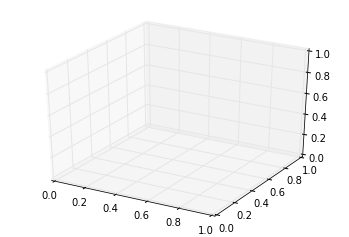## Heston Model Calibration

Heston model is defined by the following stochastic differential equations.

\begin{eqnarray} dS(t, S) &=& \mu S dt + \sqrt{v} S dW_1 \\ dv(t, S) &=& \kappa (\theta - v) dt + \sigma \sqrt{v} dW_2 \\ dW_1 dW_2 &=& \rho dt \end{eqnarray}

Here the asset is modeled as a stochastic process that depends on volatility $v$ which is a mean reverting stochastic process with a constant volatility of volatility $\sigma$. The two stochastic processes have a correlation $\rho$.

Let us look at how we can calibrate the Heston model to some market quotes. As an example, let's say we are interested in trading options with 1 year maturity. So we will calibrate the Heston model to fit to market volatility quotes with one year maturity. Before we do that, we need to construct the pricing engine that the calibration routines would need. In order to do that, we start by constructing the Heston model with some dummy starting parameters as shown below.

In :
# dummy parameters
v0 = 0.01; kappa = 0.2; theta = 0.02; rho = -0.75; sigma = 0.5;

process = ql.HestonProcess(flat_ts, dividend_ts,
ql.QuoteHandle(ql.SimpleQuote(spot)),
v0, kappa, theta, sigma, rho)
model = ql.HestonModel(process)
engine = ql.AnalyticHestonEngine(model)
# engine = ql.FdHestonVanillaEngine(model)


Now that we have the Heston model and a pricing engine, let us pick the quotes with all strikes and 1 year maturity in order to calibrate the Heston model. We build the Heston model helper which will be fed into the calibration routines.

In :
heston_helpers = []
black_var_surface.setInterpolation("bicubic")
one_year_idx = 11 # 12th row in data is for 1 year expiry
date = expiration_dates[one_year_idx]
for j, s in enumerate(strikes):
t = (date - calculation_date )
p = ql.Period(t, ql.Days)
sigma = data[one_year_idx][j]
#sigma = black_var_surface.blackVol(t/365.25, s)
helper = ql.HestonModelHelper(p, calendar, spot, s,
ql.QuoteHandle(ql.SimpleQuote(sigma)),
flat_ts,
dividend_ts)
helper.setPricingEngine(engine)
heston_helpers.append(helper)

In :
lm = ql.LevenbergMarquardt(1e-8, 1e-8, 1e-8)
model.calibrate(heston_helpers, lm,
ql.EndCriteria(500, 50, 1.0e-8,1.0e-8, 1.0e-8))
theta, kappa, sigma, rho, v0 = model.params()

In :
print "theta = %f, kappa = %f, sigma = %f, rho = %f, v0 = %f" % (theta, kappa, sigma, rho, v0)

theta = 0.135147, kappa = 1.878471, sigma = 0.002035, rho = -1.000000, v0 = 0.043485


Let us look at the quality of calibration by pricing the options used in the calibration using the model and lets get an estimate of the relative error.

In :
avg = 0.0

print "%15s %15s %15s %20s" % (
"Strikes", "Market Value",
"Model Value", "Relative Error (%)")
print "="*70
for i, opt in enumerate(heston_helpers):
err = (opt.modelValue()/opt.marketValue() - 1.0)
print "%15.2f %14.5f %15.5f %20.7f " % (
strikes[i], opt.marketValue(),
opt.modelValue(),
100.0*(opt.modelValue()/opt.marketValue() - 1.0))
avg += abs(err)
avg = avg*100.0/len(heston_helpers)
print "-"*70
print "Average Abs Error (%%) : %5.3f" % (avg)

        Strikes    Market Value     Model Value   Relative Error (%)
======================================================================
527.50      184.23863       177.26114           -3.7872037
560.46      162.13295       156.75990           -3.3139776
593.43      141.96233       138.13403           -2.6967003
626.40      123.03554       121.33552           -1.3817289
659.37      108.50169       106.27965           -2.0479306
692.34       93.29771        92.86049           -0.4686261
725.31       79.64951        80.95876            1.6437604
758.28       67.62146        70.44848            4.1806550
----------------------------------------------------------------------
Average Abs Error (%) : 2.440


 Mathias R. Fengler, Arbitrage Free Smoothing of Implied Volatility Surface, https://core.ac.uk/download/files/153/6978470.pdf